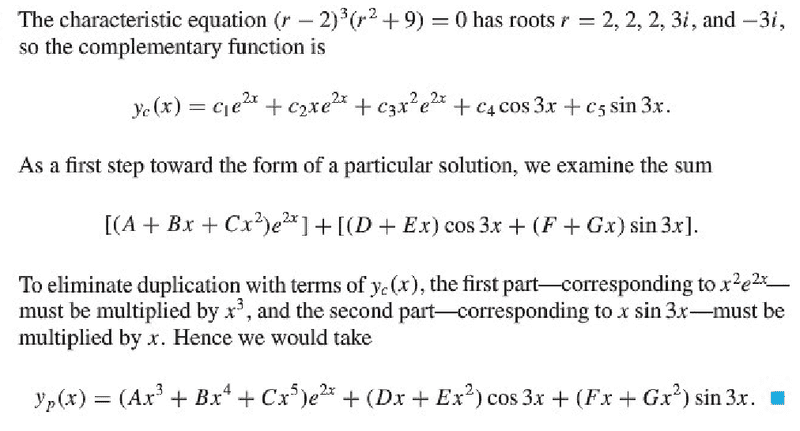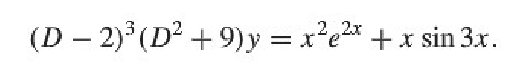# Need help understanding an aspect of undetermined coeff'sI am comfortable with the need to multiply the polynomial in front of $e^{2x}$ by $x^3$, that makes perfect sense in terms of what the text has already said about how no term in the particular solution should duplicate a term in the complementary solution, and that itself is logical in terms of the linear independence of the solutions.

What I don't fully understand is the need to multiply the polynomials in front of the trig functions by $x$.

$(D+Ex)\cos{3x} + (F+Gx)\sin{3x}$ already has non-duplicated terms in it, ie, $Ex\cos{3x}$ and $Gx\sin{3x}$.

Why wouldn't it be sufficient to simply use $(D+Ex)\cos{3x} + (F+Gx)\sin{3x}$ and assume that D and F will be zero or else absorbed by linearity into the undetermined constant coefficients of the corresponding trig functions in the complementary solution?

I have of course tried this, and it turns out that indeed the $x\cos{3x}$ and $x\sin{3x}$ terms cancel out when substituted back into the original differential equation, which isI've subbed the trial solution $(D+Ex)\cos{3x} + (F+Gx)\sin{3x}$ into $(D^2+9)y$ (the factor in the diff eq that produces the trig components of the RHS), and I can see how in this instance the $x\cos{3x}$ and $x\sin{3x}$ terms cancel out, leaving no $x\sin{3x}$ term.

I have come to agree with the insufficiency of $(D+Ex)\cos{3x} + (F+Gx)\sin{3x}$ in this particular case, but I don't know why or how this carries over to the general case.

Why wouldn't $(D+Ex)\cos{3x} + (F+Gx)\sin{3x}$ generally be okay in the particular solution when no $x\sin{3x}$ or $x\cos{3x}$ terms appear in the complementary solution?

HallsofIvy
Homework Helper
kostoglotov said:
What I don't fully understand is the need to multiply the polynomials in front of the trig functions by $x$.

$(D+Ex)\cos{3x} + (F+Gx)\sin{3x}$ already has non-duplicated terms in it, ie, $Ex\cos{3x}$ and $Gx\sin{3x}$.

Why wouldn't it be sufficient to simply use $(D+Ex)\cos{3x} + (F+Gx)\sin{3x}$ and assume that D and F will be zero or else absorbed by linearity into the undetermined constant coefficients of the corresponding trig functions in the complementary solution?
Because sin(3x) and cos(3x) are part of the solution to the homogeneous equation, "Ax sin(3x)+ Bx cos(3x)" would give a solution to an equation with non-homogeneous part "sin(3x)+ cos(3x)". In order to get a solution with non-homogeneous part "x sin(3x)+ x cos(3x)" you have to multiply by x yet again, using $x^2 sin(3x)+ x^2 cos(3x)$. In general, if you have a non-homogenous part $$x^n sin(3x)$$ and/or $$x^n cos(3x)$$ you need to use $$Ax^{n+1} sin(3x)+ Bx^{n+1} cos(3x)$$.

Last edited by a moderator: